# CHSL (10+2) Model Papers Mock Test -5 Arithmetic

## CHSL (10+2) Model Papers Mock Test -5 Arithmetic

CHSL (10+2) Model Papers Mock Test -5 Arithmetic

1.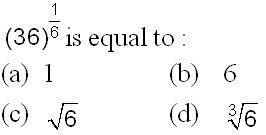2. A common factor of (137 + 117) and (135 + 115) is :

(a) 24
(b) 135 + 115
(c) 132 + 112
(d) None of the above

3. If log10 2 = 0.3010 is given, then log2 10 is equal to: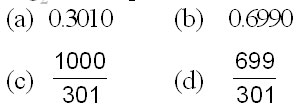4.(a) 0.02
(b) 0.004
(c) 0.4
(d) 0.04

5. The next number of the sequence 3, 5, 9, 17, 33 … is:

(a) 65
(b) 60
(c) 50
(d) 49

6.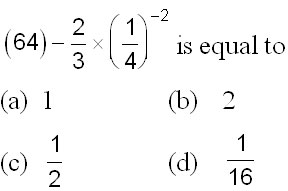7. The H.C.F. of two numbers is 8. Which one of the following can never be their L.C.M.?

(a) 24
(b) 48
(c) 56
(d) 60

8. If the sum of two numbers is 22 and the sum of their squares is 404, then the product of the numbers is:

(a) 40
(b) 44
(c) 80
(d) 88

9.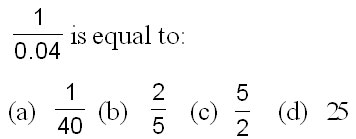10.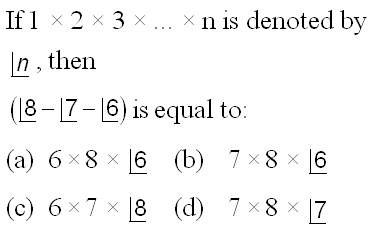11. The sum of first 20 odd natural numbers is equal to :

(a) 210
(b) 300
(c) 400
(d) 420

12. simplifies to :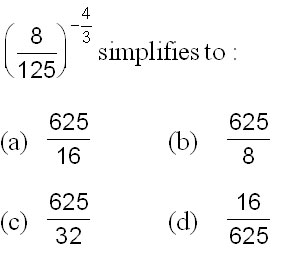13. (6.5 × 6.5 – 45.5 + 3.5 × 3.5) is equal to :

(a) 10
(b) 9
(c) 7
(d) 6

14. What number should be subtracted from both terms of the ratio 15 : 19 in order to make it 3 : 4?

(a) 9
(b) 6
(c) 5
(d) 3

15. If p : q = r : s = t : u = 2 : 3, then (mp + nr + ot) : (mq + ns + ou) is equal to :

(a) 1 : 3
(b) 1 : 2
(c) 2 : 3
(d) 3 : 2

16. If a : b = c : d = e : f = 1 : 2, then (pa + qc + re) : (pb + qd + rf) is equal to

(a) p : (q + r)
(b) (p + q) : r
(c) 2 : 3
(d) 1 : 2

17. If x : y = 3 : 1, then x3 – y3 : x3 + y3 = ?

(a) 13 : 14
(b) 14 : 13
(c) 10 : 11
(d) 11 : 10

18. If 10% of m is the same as 20% of n, then m : n is equal to

(a) 2 : 1
(b) 1 : 2
(c) 1 : 10
(d) 1 :20

19. How many sides does a regular polygon have whose interior and exterior angles are in the ratio 2 : 1?

(a) 3
(b) 5
(c) 6
(d) 12

20.The area (in sq.cm.) of the largest circle that can be drawn inside a square of side 28 cm, is

(a) 17248
(b) 784
(c) 8624
(d) 616

21.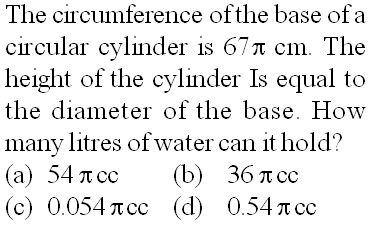22.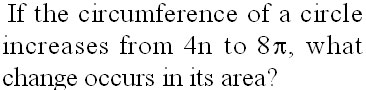(a) It doubles
(b) It triples
(d) It is halved

23. A starts business with Rs. 3500/- and after 5 months, B joins with A as his partner. After a year, the profit is divided in the ratio 2 : 3. What is B’s contribution in the capital?

(a) Rs. 8000/­
(b) Rs. 8500/­
(c) Rs. 9000/­
(d) Rs. 7500/­

24. A, B and C rent a pasture. A puts in 10 oxen for 7 months, B 12 oxen for 5 months and C 15 oxen for 3 months for grazing. If the rent of the pasture is Rs.175/-, how much must C pay as his share of rent?

(a) Rs. 45/-
(b) Rs. 50/­
(c) Rs.55/-
(d) Rs. 60/­

25. A reduction of 20% in the price of oranges enables a man to buy 5 oranges more for Rs. 10/-. The price of an orange before reduction was:

(a) 20 paise
(b) 40 paise
(c) 50 paise
(d) 60 paise

26. In an examination, a student who gets 20% of the maximum marks fails by 5 marks. Another student who scores 30% of the maximum marks gets 20 marks more than the pass marks. The necessary percentage required for passing is

(a) 32%
(b) 23%
(c) 22%
(d) 20%

27. When 60 is subtracted from 60% of a number, the result is 60. The number is

(a) 120
(b) 150
(c) 180
(d) 200

28.  :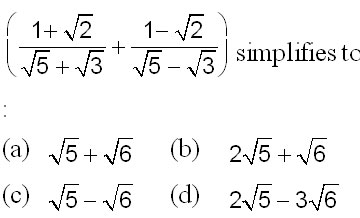29.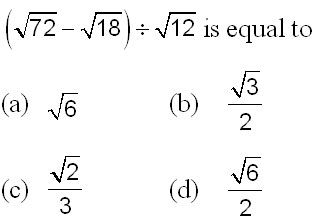30.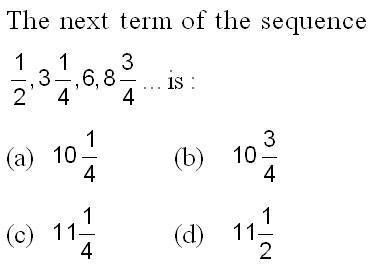31.32. If 17200 is divided by 18, the remainder is :

(a) 17
(b) 16
(c) 1
(d) 2

33. On simplification 3034 – (1002 ÷ 20.04) is equal to

(a) 3029
(b) 2984
(c) 2993
(d) 2543

34.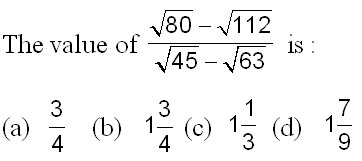35. When simplified, the expression – is equal to :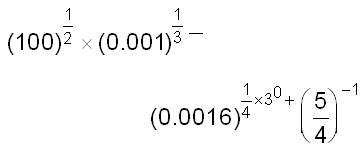36.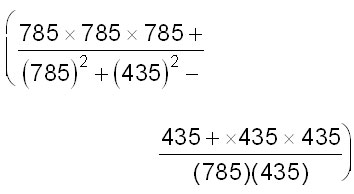(a) 350
(b) 785
(c) 1220
(d) 1320

37. Find the missing number of the sequence : “3, 14, 25, 36, 47 , ?”

(a) 1114
(b) 1111
(c) 1113
(d) None of these

38.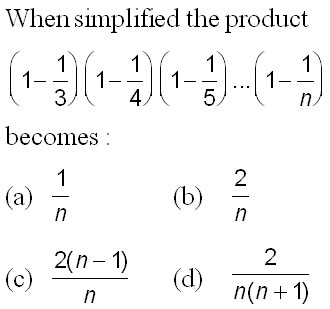39. The sum (101 + 102 + 103 + … + 200) is equal to :

(a) 15000
(b) 15025
(c) 15050
(d) 25000

40. The sum of all natural numbers from 75 to 97 is:

(a) 1598
(b) 1798
(c) 1958
(d) 1978

41.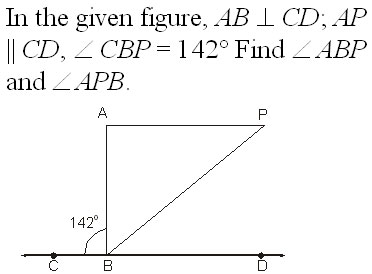(a) 52°, 38°
(b) 56°, 34°
(c) 51°, 39°
(d) 57°, 33°

42.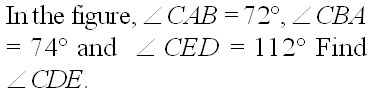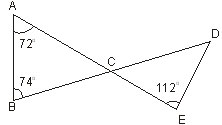(a) 34°
(b) 33°
(c) 35°
(d) 38°

43. The point of intersection of the line x + y + 1 = 0 and 2x – y + 5 = 0 is

(a) (–1, 1)
(b) (–2, 1)
(c) (1, 2)
(d) (1, –2)

44 The equation of the line passing through the point of intersection of the lines 5x – 2y = 3, 4x – 7y + 3 = 0 and parallel to the lines 3x – 2y + 5 = 0

(a) 3x – 2y = 0
(b) 3x – 2y =1
(c) 3x – 2y = 5
(d) None of these

45 The equation of a straight line passing through the intersection of the lines x + y + 1 = 0, 2x – y + 5 = 0 and through the point (5, –2).

(a) 3x + 5y = 1
(b) 3x – 7y =1
(c) 3x + 7y = 1
(d) None of these

46. The equation of a line parallel to 3x – 2y + 1 = 0 and passing through the origin is

(a) 3x – 2y = 0
(b) 3x – 2y = 5
(c) 3x – 2y = 1
(d) None of these

47.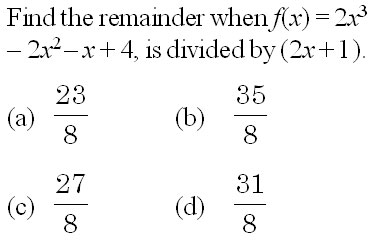48. If the polynomials f(x) = x3 – ax2 + 9x + a is divided by (x – a).

(a) 10a
(b) 6a
(c) 7a
(d) 5a

49. If x2 – 1 is a factor of the polynomial f(x) = 2x2 + Ax2 + Bx + 3, then find A and B.

(a) –3,2
(b) –3, –2
(c) 3,–2
(d) Can’t be determined

50. When 4x3 – ax2 + bx – 4 is divided by x – 2 and x + 1, the respective remainders are 20 and –13. Find the values of a and b

(a) –3, –2
(b) –3,2
(c) 3, –2
(d) 3, 2